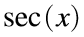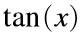# Problem 46034. Construct the Seidel-Entringer-Arnold triangle

Several problems in Cody ask us to construct part or all of triangles in which entries follow a pattern. Cody Problems 37, 1463, 44037, and 44904 involve Pascal's triangle, which consists of the binomial coefficients, and Cody Problem 1845 extends Pascal's triangle to a pyramid. Cody Problem 45460 involves the Bernoulli triangle, which consists of partial sums of the binomial coefficients.
This problem deals with the Seidel-Entringer-Arnold triangle (also called the Euler-Bernoulli triangle and the secant-tangent triangle). The first eight layers are
1
0 1
1 1 0
0 1 2 2
5 5 4 2 0
0 5 10 14 16 16
61 61 56 46 32 16 0
0 61 122 178 224 256 272 272
The name "secant-tangent triangle" arises because the sides contain the coefficients in the Taylor series forand:Construct the nth layer of this triangle.
Hint: Use the boustrophedon (or ox-plowing) rule.

### Solution Stats

60.61% Correct | 39.39% Incorrect
Last Solution submitted on Jun 01, 2023

### Community Treasure Hunt

Find the treasures in MATLAB Central and discover how the community can help you!

Start Hunting!